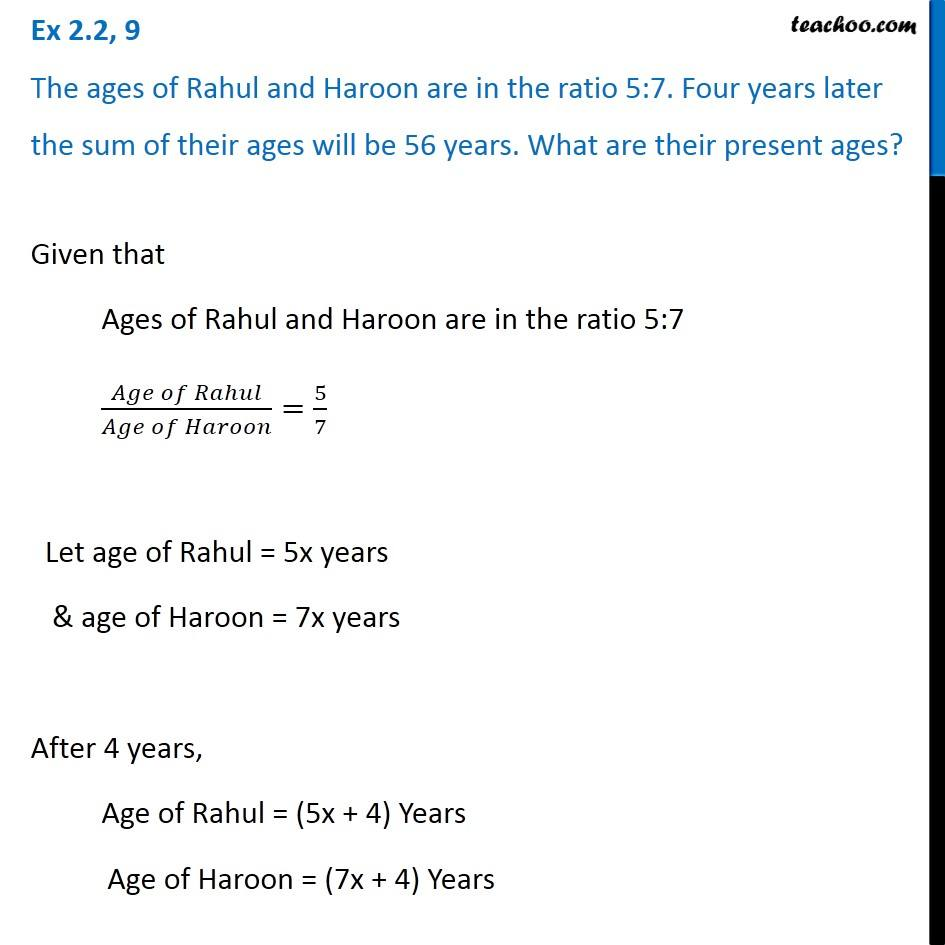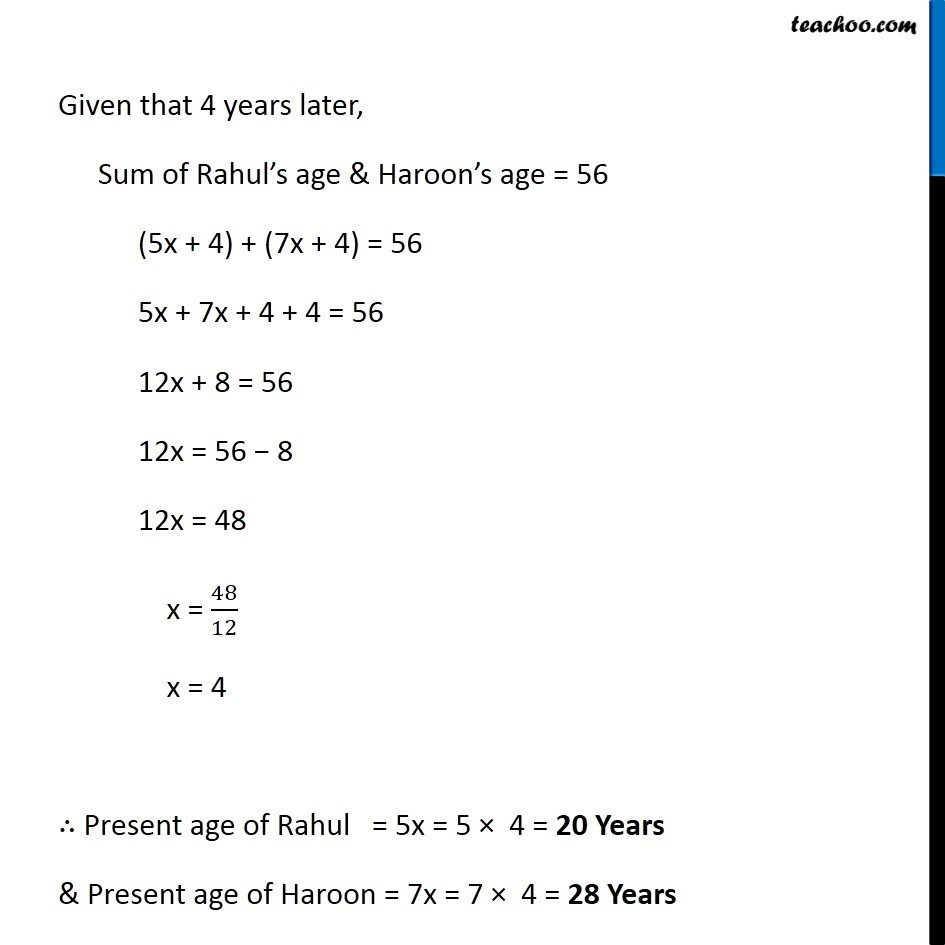Solving easy equations - Word Problems

Chapter 2 Class 8 Linear Equations in One Variable
Serial order wiseLearn in your speed, with individual attention - Teachoo Maths 1-on-1 Class

### Transcript

Question 9 The ages of Rahul and Haroon are in the ratio 5:7. Four years later the sum of their ages will be 56 years. What are their present ages?Given that Ages of Rahul and Haroon are in the ratio 5:7 (𝐴𝑔𝑒 𝑜𝑓 𝑅𝑎ℎ𝑢𝑙)/(𝐴𝑔𝑒 𝑜𝑓 𝐻𝑎𝑟𝑜𝑜𝑛)=5/7 Let age of Rahul = 5x years & age of Haroon = 7x years After 4 years, Age of Rahul = (5x + 4) Years Age of Haroon = (7x + 4) Years Given that 4 years later, Sum of Rahul’s age & Haroon’s age = 56 (5x + 4) + (7x + 4) = 56 5x + 7x + 4 + 4 = 56 12x + 8 = 56 12x = 56 − 8 12x = 48 x = 48/12 x = 4 ∴ Present age of Rahul = 5x = 5 × 4 = 20 Years & Present age of Haroon = 7x = 7 × 4 = 28 Years